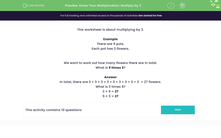# Multiply by 3, using repeated addition

In this worksheet, students learn to multiply by 3.Key stage:  KS 2

Curriculum topic:   Number: Multiplication and Division

Curriculum subtopic:   Use Multiplication/Division Facts (3, 4 and 8)

Difficulty level:#### Worksheet Overview

In this activity, we will learn about multiplying by 3.

To multiply by three, we can use repeated addition.

Here are 3 cats in a group.Let's think about what happens if we have 4 groups of 3 cats and we want to find the total!We could count the cats one by one but this would take a while.

4 groups of 3 looks like this as repeated addition

3 + 3 + 3 + 3 = 12

There are 12 cats in total!

Now let's try an example of multiplying by 3, using repeated addition.

Example

There are 9 pots.

Each pot has 3 flowers.We want to work out how many flowers there are in total.

What is 9 times 3?

In total, there are 3 + 3 + 3 + 3 + 3 + 3 + 3 + 3 + 3  = 27 flowers.

What is 3 times 9?

3 × 9 = 27

9 × 3 = 27

Now that you understand repeated addition, let's try some more questions.

### What is EdPlace?

We're your National Curriculum aligned online education content provider helping each child succeed in English, maths and science from year 1 to GCSE. With an EdPlace account you’ll be able to track and measure progress, helping each child achieve their best. We build confidence and attainment by personalising each child’s learning at a level that suits them.

Get started